## A mass weighing 32 pounds stretches a spring 2 feet. Determine the amplitude and period of motion if the mass is initially released from a p

Question

A mass weighing 32 pounds stretches a spring 2 feet. Determine the amplitude and period of motion if the mass is initially released from a point 1 foot above the equilibrium position with an upward velocity of 6 ft/s.

in progress 0
5 months 2021-08-22T05:58:17+00:00 1 Answers 67 views 0

## Answers ( )

1. A mass weighing 32 pounds stretches a spring 2 feet.

(a) Determine the amplitude and period of motion if the mass is initially released from a point 1 foot above the equilibrium position with an upward velocity of 6 ft/s.

(b) How many complete cycles will the mass have completed at the end of 4 seconds?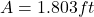Period =seconds

8 cycles

Explanation:

A mass weighing 32 pounds stretches a spring 2 feet;

it implies that the mass (m) =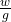m=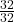= 1 slug

Also from Hooke’s Law

2 k = 32

k =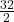k = 16 lb/ft

Using the function: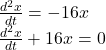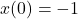(because of the initial position being above the equilibrium position)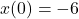( as a result of upward velocity)

NOW, we have: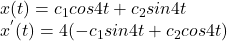However;means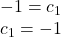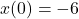also implies that: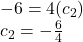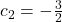Hence,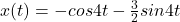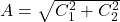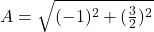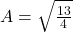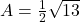Period can be calculated as follows:

=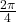=seconds

How many complete cycles will the mass have completed at the end of 4 seconds?

At the end of 4 seconds, we have: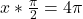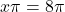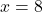cycles